Topic: Charts & Graphs

Free Printable Worksheets Using Charts and Graphs

Reading a Calendar Worksheet – C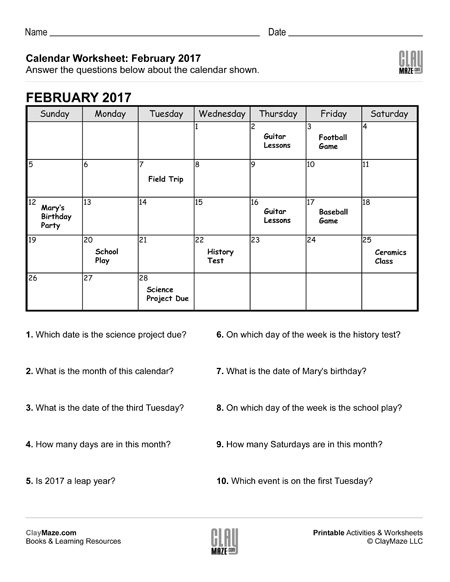This worksheet is for practice reading a calendar. Below the calendar are questions about the calendar, weekdays, dates and the events displayed....

Reading a Calendar Worksheet – DThis worksheet features a calendar with events posted on different dates. Following that are questions about the calendar, dates, weekdays and events listed....

General Statistics Worksheet – A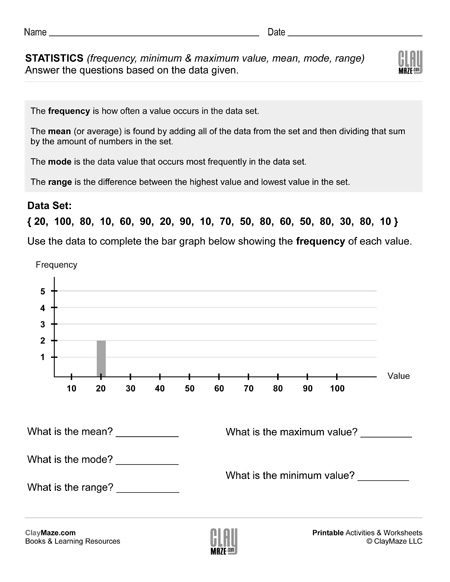This worksheet has a few statistics terms defined at the top. Following that is a data set and a graph to fill out using the data given. Below that are questions about the data which use the...

General Statistics Worksheet – BThere are statistics terms defined at the top of this worksheet followed by a data set and a graph to be filled out using the data set. Then the student asked questions about the data using the...

General Statistics Worksheet – C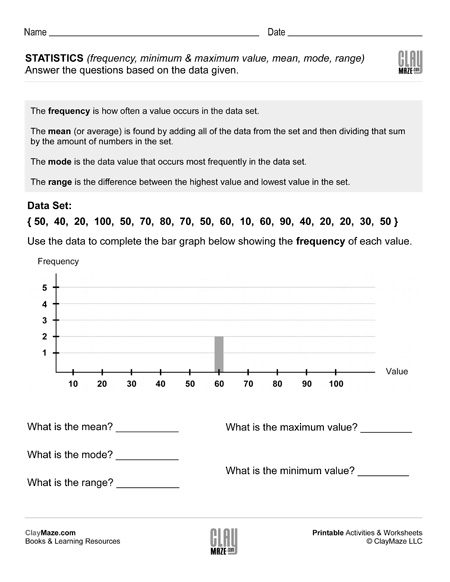The worksheet has statistics terms defined at the top for reference. Below that is a set of data values. Using these values, the student is asked to complete the graph. Following the graphs are...

General Statistics Worksheet – DThis worksheet gives a data set that should be used to complete the graph and answer the questions. The student can refer to the statistics terms defined at the top of the worksheet to answer the...

Counting Chart 1 to 100 (set 1)1 to 100 number chart worksheet for kindergarten and first grade. In this worksheet the student is asked to fill in the missing numbers from 1 to 100. Half of them are filled in....

Counting Chart 1 to 100 (set 2)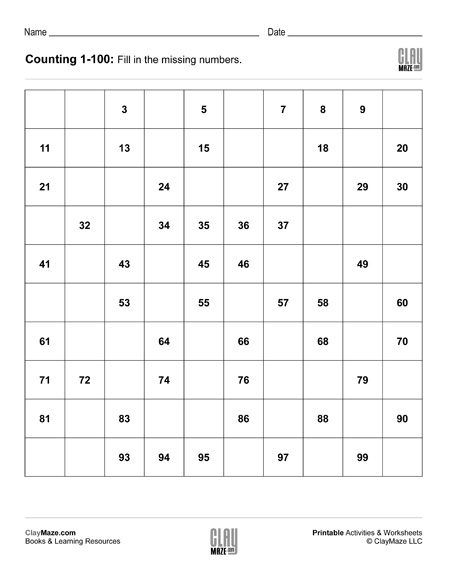Kindergarten and first grade counting worksheet for the numbers 1 through 100. Half of the numbers are missing in the chart and the student is asked to fill in those missing numbers....

Counting Chart 1 to 100 (set 3)Kindergarten and elementary school number chart worksheet for the numbers 1 to 100. The student is asked to fill in the missing number counting by 1’s....

Counting Chart 1 to 100 (set 4)Counting worksheet: Numbers 1 to 100. Fill in the missing numbers. Free and printable number chart worksheet....

Skip Counting Worksheet – Counting by 2’s and Counting by 5’s (blank chart)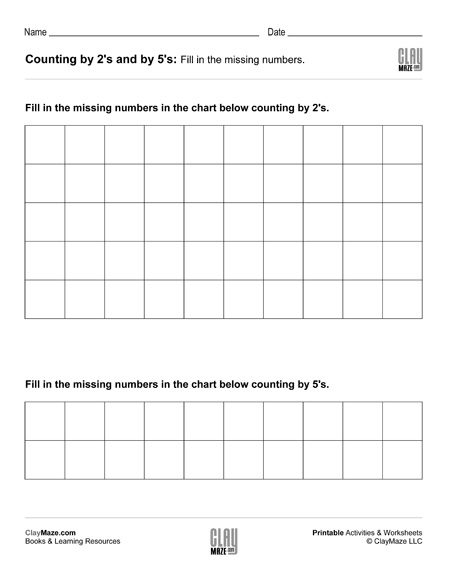Free and printable blank skip counting number chart for the numbers up to 100....

Skip Counting Worksheet – Counting by 2’s and Counting by 5’s (Set 1)Up 100 skip counting number charts worksheet. The student is asked to fill in the missing numbers up to 100 counting by 2’s and counting by 5’s....

Skip Counting Worksheet – Counting by 2’s and Counting by 5’s (Set 2)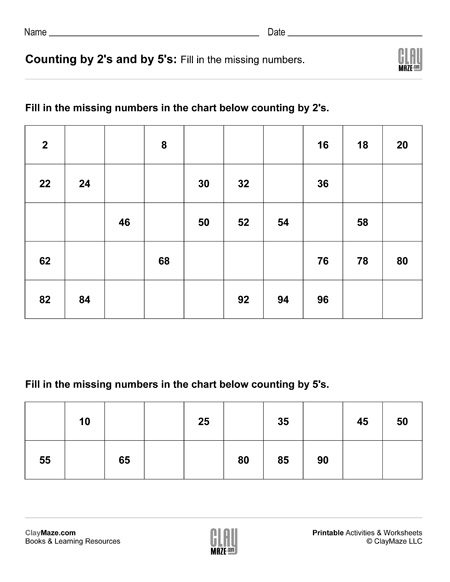Skip counting worksheet for numbers up to 100. Count by 2’s to fill in the first chart and count by 5’s to fill in the second....

Skip Counting Worksheet – Counting by 2’s and Counting by 5’s (Set 3)Skip counting worksheet with 2 charts. Counting by 5’s and counting by 2’s....

Skip Counting Worksheet – Counting by 2’s and Counting by 5’s (Set 4)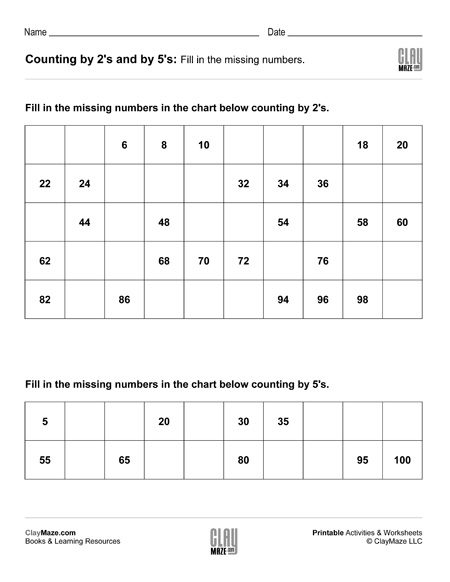Skip counting worksheet – Numbers up to 100. Fill in the missing numbers counting by 2’s and counting by 5’s....